# The wave nature of light

## The wave nature of light

The following text is used only for teaching, research, scholarship, educational use and informative purpose following the fair use principles.

We thank the authors of the texts and the source web site that give us the opportunity to share their knowledge

# The wave nature of light

The English Physicist Thomas Young proved that light was made of waves.*
To Demonstrate the Wave nature of Light (Young’s Slits Experiment)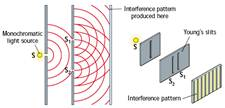• Set up the equipment as shown. S is a monochromatic light source (light of one wavelength only).
• Light from S shines onto the first narrow slit. It undergoes diffraction here and illuminates the slits S1 and S2.

These two slits are known as Young’s Slits.

• Diffraction occurs at each of these slits; in the region between them (where light from each overlaps) constructive and destructive interference occurs.
• The result is that a series of bright lines are seen either on a screen or through a spectrometer.

• Conclusion: The fact that light undergoes Interference tells us that light travels as a wave.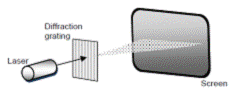Interference Colours can be seen on petrol films and soap bubbles, due to the interference of light waves which have been reflected from the different interfaces.

The Diffraction Grating

A diffraction grating consists of a piece of transparent material on which a very large number of opaque (black) parallel lines are engraved*.

The distance between two adjacent slits is referred to as ‘the slit width’ or ‘the grating constant’. its symbol is d.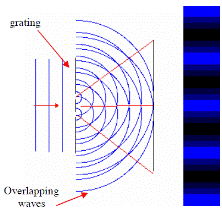In general, if a grating has n lines per mm Þ  d = 1/n millimetres
or
if a grating has n lines per m
Þ  d = 1/n metres
Note: you will often be told in a question that the grating has n lines per mm,
so multiply  by 1000 to get the number of lines per metre, then just invert to get d, the grating constant.
Now try Questions 3 and 4 page 212.

Formula for a diffraction grating
nl = d Sin q
n = order (first order, second order etc.)
l = wavelength
d = distance between lines (slit width)
q = angle between straight through position and the order in question.
See Worked Problems 1 – 4, page 212. Then try Exercise 18.1, page 212.Derivation of Formula nl = d Sin q*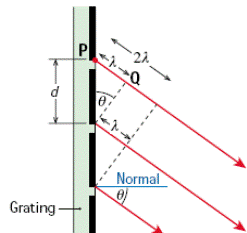From the diagram we can see that
(i)         For constructive interference to occur, the extra path length that the top ray travels must be an integer number of wavelengths (nl)                                                                                      {Eqn (1)}

(ii)       Using trigonometry, this extra path length is equal to d sin q, where d is the slit width                                             {Eqn (2)}

Equating (1) and (2) gives us nl = d Sin q

Polarisation*
A Polarised wave is a wave which vibrates in one plane only.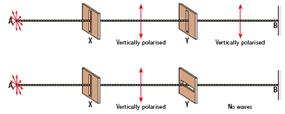• The diagram shows an unpolarised wave on the left (it vibrates in all directions) and two polarising filters.
• The first filter will only allow waves which are vertically polarised to pass through it.
• These waves will only pass through a second Polaroid if the second polaroid is parallel to that of the first.

To Demonstration Polarisation using two polaroids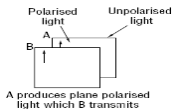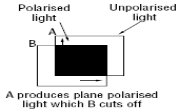• Light from an incandescent source (something which emits light when heated) is unpolarised, i.e. the electric and magnetic fields are oscillating in many different planes.
• If light from such a source is passed through a substance called a Polaroid the emerging rays are now polarised, i.e. oscillate in one plane only.
• If this light is then passed through a second polaroid, it only gets through if the second polaroid is parallel to that of the first.
• If the second polaroid is then rotated through 900, no light gets through.

Note: only transverse waves can be polarised so the fact that light can be polarised shows that light is a transverse wave. *

Applications: Sunglasses, Stress Polarisation (used to detect faults or stresses in materials)

Dispersion
Dispersion is the separating out of the different colours present in white light.
Dispersion can be brought about by either a prism or a diffraction grating.

Dispersion is the principle behind the array of colours seen in rainbows, polished gemstones and on surface of CDs.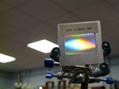Dispersion due to a Diffraction Grating
A Diffraction Grating causes dispersion because from the formula nl = d sin q, if l is different, q will be different,
i.e. different colours are diffracted by different amounts.

From this we can see that the colour with the largest wavelength (Red) gets deviated the most.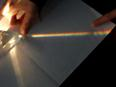Dispersion due to a Prism
A Prism causes dispersion because the Refractive Index of the medium is slightly different for different wavelengths, therefore each wavelength gets refracted (bent) by a different amount.
In this case Blue gets deviated the most*.

Recombination
If a given prism is used to disperse white light, a second identical – inverted - prism can be used to recombine the components back into white light
.
Primary and Secondary Colours
Primary Colours
The primary colours are three colours such that when combined in equal intensity produce white light.
The three primary colours are Red, Green and Blue*.

Secondary Colours
When two primary colours are mixed in equal intensity, the colour formed is a secondary colour.
Yellow, Cyan and Magenta are the three secondary colours.
Complementary Colours
Complementary colours are pairs of colours consisting of a primary and a secondary colour, such that when combined they give white light*
.
The fact that any given colour can be produced from a combination of the three primary colours means that only these three coloured-bulbs are needed in televisions or in Stage Lighting kits.

The Electromagnetic Spectrum*
You are expected to know the relative positions of the different radiations in terms of their frequency and wavelength.

Frequency Increasing Þ

 Radio Micro Infrared Visible Ultraviolet X-ray Gamma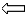Wavelength Increasing

The colours of visible light (in order of increasing frequency) are Red, Orange, Yellow, Green, Blue, Indigo, Violet. [Make up your own mnemonic, but you must remember that Red has the lowest frequency and Blue one of the highest].
In practice the colour we see is governed by the physiology of the brain. It was actually Thomas Young who was the first to realise this also. He was quite a dude.

Relationship between Wavelength and Colour
While we might say that yellow has a wavelength of 7 × 10-7 metres (often written as 700 nm), it would be more correct to say that yellow covers a range of wavelengths and gradually cedes to orange on one side and green on the other.

Characteristics

• Is an electromagnetic wave
• Causes objects to fluoresce
• Can be detected by photographic plating

Characteristics

• Is an electromagnetic wave
• Can be detected with a heat-sensitive camera e.g. ‘night-vision’ cameras.

Applications of Infra-Red technology
Infra-red camera (used in Night-Vision goggles)
Medical Applications: Studies have shown that an abnormal infrared image is the single most important marker of high risk for developing breast cancer.
The Greenhouse Effect
The Earth receives energy from the Sun in the form of radiation, most of which is in the visible wavelength region and which passes through the Earth’s atmosphere on the way to the planet’s surface. Some of this radiation then gets reflected (re-radiated) off the surface of the Earth as infra-red radiation. Much of this radiation, which would otherwise radiate back out to space, gets absorbed by the atmosphere (by carbon dioxide, water vapour and methane) and as a result the atmosphere heats up.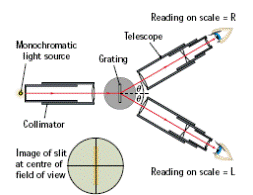The Spectrometer and the function of its parts
Collimator: To ensure that the light which comes out (onto the diffraction grating) is a parallel beam.

Astronomical Telescope: The telescope is used to view an image of the slit.
Cross-Threads: Used to centre the slit

Mandatory Experiment:
To Measure the wavelength of Light

Leaving Cert Physics Syllabus

 Content Depth of Treatment Activities STS 1. Diffraction and interference Use of diffraction grating formula: nl = d Sin q Derivation of formula Suitable method of demonstrating the wave nature of light. Appropriate calculations. Interference colours Petrol film, soap bubbles. 2. Light as a transverse wave motion Polarisation Demonstration of polarisation using polaroids or other method. Stress polarisation. Polaroid sungalsses. 3. Dispersion Dispersion by a prism and a diffraction grating. Recombination by a prism. Demonstration. Rainbows, polished gemstones. Colours seen on surfaces of compact discs. 4. Colours Primary, secondary, complementary colours. Addition of colours. Pigment colours need not be considered. Demonstration. Stage lighting, television. 5. Electromagnetic Spectrum Relative positions of radiation in terms of wavelength and frequency. Detection of UV and IR radiation. Demonstration. Ultraviolet and ozone layer. Infrared camera: Medical applications Night vision. Greenhouse effect. 6. The spectrometer The spectrometer and the function of its parts. Demonstration. Experiment: Measurement of the wavelength of monochromatic light.

MEASUREMENT OF THE WAVELENGTH OF MONOCHROMATIC LIGHT

APPARATUS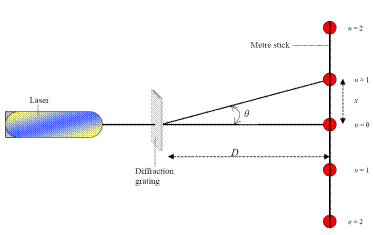Laser, diffraction grating (600 lines per mm), 2 metre sticks.

DIAGRAM

PROCEDURE

• Clamp a metre stick horizontally in a stand.
• Allow the laser beam to hit the metre stick normally (at 90°).

• Move the metre stick sideways until the spot is on the 50 cm mark.
• Place the grating between the laser and the metre stick, at right angles to the beam.

• Observe the interference pattern on the metre stick – a series of bright spots.
• Calculate the mean distance x between the centre (n=1) bright spot and the first (n =1) bright spot on both sides of centre.

• Measure the distance D from the grating to the metre stick.
• Calculate θ using tan θ = x/D.

• Calculate the distance d between the slits, using d =1/N, where N is the number of lines per metre on the grating.
• Calculate the wavelength λ using nλ = dsinθ.

• Repeat this procedure for different values of n and get the average value for λ.

RESULTS

 n x (m) D (m) θ λ

CONCLUSION
We got a value of 7.10 × 10-7 m, which sits nicely in the accepted range of 620 – 750 nm for red light. Job is oxo

SOURCES OF ERROR / PRECAUTIONS

• The diffraction grating may not have been exactly at right angles to the beam of light; x should be the same on both sides. Measure x on both sides of the n = 0 position and take the average of the two readings. To reduce the percentage error further just measure the total distance between both points and divide by 2
• Determining the exact middle of the dot on the screen was difficult (repeat and get an average)

NOTE
A nice variation on this is to take a school laser into a large darkened room (e.g. a gym) and get very large distances for D and x on either side.

Extra Credit
*The English Physicist Thomas Young proved that light was made of waves.
One reason scientists found it difficult to believe that light was made of waves was because they figured that there needed to be a medium, but couldn’t figure out what the medium in space would be. So they came up with an imaginary medium, which they called Aether.
There is an ethereal medium pervading all bodies. The parts of this medium are capable of being set in motion by electric currents and magnets.
James Clerk Maxwell.
It was Maxwell (one of the greatest scientists of the 19th century) who was finally able to show mathematically how Electricity and Magnetism were interconnected.
You don’t hear too much about aether these days – I think perhaps scientists are a little embarrassed when they think about it and would rather just forget all about their little boo-boo.

Why is there a slit in front of the double slit?
I’m not 100% certain, but my guess is that the width of the first slit matches the wavelength of one form of visible light, so now only single wavelength light reaches the second set of slits.

*The diffraction grating
Students often have difficulty believing that an apparently clear piece of plastic can have 1,000 lines per cm etched on it.
It is no harm to remind yourselves that a compact disc can also act as a diffraction grating if laser light is shone on it, even though you can’t see the etchings in those either (although in this case the light doesn’t actually pass through the c.d.)
The spaces between the lines in a grating behave as slits and allow the light to pass through and diffract. The light which passes through then behaves as individual waves, which interfere both constructively and destructively.

*Derivation of formula nl = dSinq
This derivation is very short to write, but each line involves quite an amount of (sometimes tricky) concepts.

• Understanding that the extra path wavelength travelled by the upper wave is an integer number of wavelengths greater than the lower waves, for constructive interference to occur.
• Understanding that the angle q on top is equal to the angle q at the bottom.
• Understanding that the extra path length is represented by the opposite side of a right-angled triangle, and therefore is equal to (d)(Sin q).

Each of these concepts needs to be teased out.

*The fact that light can be polarised shows that light is a transverse wave.
Polarisation was really stumping scientists in the mid 19th century, and investigation into this topic led to important developments in the understanding of light itself. Our treatment of it here is very shallow indeed.
A short interesting history of the topic is to be found in Bill Bryson’s book, “A Short History of Nearly Everything”
*In this case Blue gets deviated the most.
You don’t need to know why, but you might want to prove it for yourself, using the relationship between R.I. and speed of the waves, and also the relationship between c, f and l.

*The three primary colours are Red, Green and Blue.
These primary colours are different from the primary colours associated with pigments or paints.
By the way, a question which troubled philosophers for a long time is whether it is possible that what you see as green, everyone one else sees as orange, and vice versa. What do you think?

*Complementary colours are pairs of colours consisting of a primary and a secondary colour, such that when combined they give white light.
For example if Blue (primary) is mixed with Yellow (secondary), the result is white light. But this shouldn’t be too surprising because Yellow is nothing more than Green and Red combined, so indirectly the three primary colours are being combined.

*The Electromagnetic Spectrum
A mnemonic for remembering the order of the EM Spectrum
(radio, micro, infrared, visible, ultraviolet, x-ray, gamma).
Randy Men Inject Viagra Until eXplosive Growth.

Ten years ago McDonalds replaced all their normal light-bulbs in their toilets with Ultra-Violet bulbs.
Apparently peoples’ veins are not visible under UV and therefore addicts can’t locate them and so can’t inject themselves.

Exam Questions

•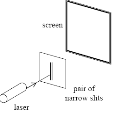Describe an experiment that demonstrates the wave nature of light.

• 

A student used a laser, as shown, to demonstrate that light is a wave motion.
Name the two phenomena that occur when the light passes through the pair of narrow slits.

•A student used a laser, as shown, to demonstrate that light is a wave motion.

• A pattern is formed on the screen. Explain how the pattern is formed.
• What is the effect on the pattern when the wavelength of the light is increased?
• What is the effect on the pattern when the distance between the slits is increased?

• [2009 OL]

In an experiment a beam of monochromatic light passes through a diffraction grating and strikes a screen.

•Describe what is observed on the screen.
• Explain, with the aid of a diagram, how this phenomenon occurs.
• What does this experiment tell us about the nature of light?
• Name the property of light that can be determined in this experiment.
• What measurements must be taken to determine the property you named?
• 

Why does diffraction not occur when light passes through a window?

• 

A sound wave is diffracted as it passes through a doorway but a light wave is not. Explain why.

• [2009 OL]

What is a diffraction grating?

• [2009 OL]

Explain the term monochromatic light.

• 

A diffraction grating has 200 lines per mm. What is the value of d in the diffraction grating formula  nλ = d sin θ ?

• 

Derive the diffraction grating formula

• 

Sound travels as longitudinal waves while light travels as transverse waves.
Explain the difference between longitudinal and transverse waves.

• 

What is meant by polarisation of waves?

• 

Which wave phenomenon can be used to distinguish between transverse waves and longitudinal waves?

• 

Describe an experiment to demonstrate that light waves are transverse waves.

• [2003 OL][2010 OL]

Give one difference between light waves and sound waves.

• [2007 OL][2010 OL]

What is meant by the term dispersion?

• [2010 OL]

Give an example of the dispersion of light occurring in nature.

• [2007 OL]

What is meant by the term spectrum?

• [2002 OL][2003 OL][2008 OL][2010 OL]

Describe an experiment to demonstrate the dispersion of white light.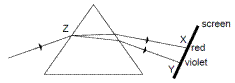• [2007 OL]
• What happens to the white light when it enters the prism at Z?
• Name the invisible radiation formed on the screen at (i) region X, (ii) region Y.
• [2003 OL][2004 OL][2007 OL]

Name two primary colours.

• [2010 OL]

Only red, green and blue lights are needed to create most lighting effects.
Explain why

•

[2007 OL]
How is a secondary colour (e.g. yellow) produced on a TV screen?

• [2003 OL]

What are complementary colours?

• [2008 OL]

Name two radiations in sunlight that the eye cannot detect.

• [2006 OL]

The table shows the relative positions of electromagnetic radiations in terms of their wavelength.

 Gamma rays A UV light IR microwaves B

Name the radiations marked A and B.

• [2006 OL]

Which one of the radiations has the shortest wavelength?

• [2003 OL]

The diagram shows a simple form of the electromagnetic spectrum, with wavelength increasing from left to right.
Copy this diagram and indicate on it the positions of the following:

 short wavelength                     →                    long wavelength gamma rays                      light                  radio waves

microwaves; infrared; ultraviolet; X-rays:

• [2002 OL][2006 OL]

Give one property which is common to all electromagnetic radiations.

• [2002 OL][2005 OL]

• [2004 OL]

Which one of the following is not part of the electromagnetic spectrum?

• 

Give two properties of radio waves.

• l [2002 OL][2006 OL][2007 OL][2008 OL]

• [2007 OL][2008 OL]

How is ultra violet radiation detected?

• [2002  OL][2006 OL]

Give two uses of microwaves.

• [2007 OL][2008 OL]

Give a use for infra red radiation.

• [2007 OL]2008 OL]

Give a use for ultra violet radiation.

• [2004 OL][2007 OL]

Give one use of X-rays.

• [2008 OL][2009 OL]

State two properties of X-rays.

• 

• 

A satellite transmits radio signals to earth. At a particular time the satellite is 1.2 × 1012 m from earth. How long does it take the signal to travel to earth?

• [2002 OL]

What is the frequency of the radio waves? The speed of light is 3 × 108 m s-1.

• 

Why are radio frequency waves not very penetrating?

• [2009 OL]

Calculate the wavelength of a radio wave whose frequency is 252 kHz. (c = f λ , c = 3.0 × 108 m s−1 )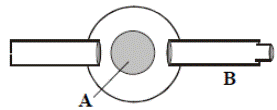• 

Name the parts labelled A and B of the spectrometer shown in the diagram.

• [2006 OL]

Give one use of a spectrometer.

• 

A person’s exposure to radiation when using a mobile phone is measured in terms of the Specific Absorption Rate (SAR). This is a measure of the rate at which radio frequency energy is absorbed by a person’s body during a phone call and is expressed in watts per kilogram.
A radio frequency wave penetrates the body to a depth that depends on its frequency. At mobile phone frequencies the wave energy is absorbed by about one centimetre of body tissue. The energy absorbed is converted into heat and is carried away by the body. Any adverse health effects from radio frequency waves are due to heating. Current scientific evidence indicates that exposure to radiation from mobile phones is unlikely to induce cancer.
(Adapted from a Dept. of Communications, Energy and Natural Resources Press Release of 22 March 2007.)

• In a three-minute phone call, 10 g of head tissue absorbs 0.36 J of radio frequency energy.
• Calculate the SAR value.
• What happens to the radio frequency energy absorbed by the body?
• Why are radio frequency waves not very penetrating?
• Give two safety precautions you should take when using a mobile phone.

Mandatory Experiments

• 
• An interference pattern is formed on a screen when green light from a laser passes normally through a diffraction grating. The grating has 80 lines per mm and the distance from the grating to the screen is 90 cm. The distance between the third order images is 23.8 cm.

Calculate the wavelength of the green light.

• Calculate the maximum number of images that are formed on the screen.
• The laser is replaced with a source of white light and a series of spectra are formed on the screen.

Explain how the diffraction grating produces a spectrum.

• Explain why a spectrum is not formed at the central (zero order) image.

• [2007 OL]

You carried out an experiment to measure the wavelength of a monochromatic light source using a diffraction grating. The diffraction grating had 600 lines per mm.

• Draw a labelled diagram of the apparatus you used.
• Name a source of monochromatic light.
• State what measurements you took during the experiment.
• What is the distance between each line on the diffraction grating?
• How did you determine the wavelength of the light?
• Give one precaution that you took to get an accurate result.

• [2004 OL]

You carried out an experiment to measure the wavelength of a monochromatic light source.

• Name a monochromatic light source.
• Draw a labelled diagram of the apparatus that you used in the experiment.
• What readings did you take during the experiment?
• What formula did you use to calculate the wavelength of the light?
• Give one precaution that you took to get an accurate result.
• 

In an experiment to measure the wavelength of monochromatic light, a diffraction pattern was produced using a diffraction grating with 500 lines per mm. The angle between the first order images was measured. This was repeated for the second and the third order images.

 Angle between first order images Angle between second order images Angle between third order images 34.20 71.60 121.60

The table shows the recorded data:

• Draw a labelled diagram of the apparatus used in the experiment.
• Explain how the first order images were identified.
• Describe how the angle between the first order images was measured.
• Use the data to calculate the wavelength of the monochromatic light.

• 

In an experiment to measure the wavelength of monochromatic light, the angle θ between a central bright image (n = 0) and the first and second order images to the left and the right was measured.
A diffraction grating with 500 lines per mm was used.
The table shows the recorded data.

 n 2 1 0 1 2 θ /degrees 36.2 17.1 0 17.2 36.3
• Describe, with the aid of a diagram, how the student obtained the data.
• Use all of the data to calculate a value for the wavelength of the light.
• Explain how using a diffraction grating with 100 lines per mm leads to a less accurate result.
• The values for the angles on the left of the central image are smaller than the corresponding ones on the right. Suggest a possible reason for this.

• 

In an experiment to measure the wavelength of monochromatic light, a narrow beam of the light fell normally on a diffraction grating. The grating had 300 lines per millimetre. A diffraction pattern was produced. The angle between the second order image to the left and the second order image to the right of the central bright image in the pattern was measured.
The angle measured was 40.60.

• Describe, with the aid of a labelled diagram, how the data was obtained.
• How was a narrow beam of light produced?
• Use the data to calculate the wavelength of the monochromatic light.
• Explain how using a diffraction grating of 500 lines per mm leads to a more accurate result.
• Give another way of improving the accuracy of this experiment.

Exam Solutions

• Shine a laser through a diffraction grating; an interference pattern will be produced on a screen, caused by interference of the light waves
• Diffraction            and Interference
•
• The slits act as sources of two coherent waves which overlap to give areas of constructive interference (bright lines) and destructive interference (dark lines).
• The pattern becomes more spread out.
•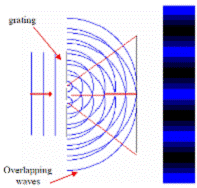The pattern becomes less spread out.
•
• A series of bright dots.
• The light waves pass through the diffraction grating and spread out on the other side after passing through the slit.

Constructive and destructive interference occurs and fringes are formed on the screen as shown in the diagram.

• Light is a wave.
• The wavelength of light can be measured.
• The distance between bright dots, distance from the screen to grating.
• The window is too wide (relative to wavelength of light).
• Diffraction only occurs when the width of the gap is approximately equal to the wavelength of the wave. This is the case for a sound wave but the wavelength of a light-wave is very small compared to the size of a doorway.
• A diffraction grating consists of a piece of transparent material on which a very large number of opaque (black) parallel lines are engraved.
• Monochromatic light is light of one wavelength only.
• d = 1/200000 = 5 × 10-6 m.
•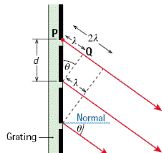From the diagram we can see that
(i)  For constructive interference to occur, the extra path length that the top ray travels must be an integer number of wavelengths (nl)                                                                                                 {Eqn (1)}
(ii) Using trigonometry, this extra path length is equal to d sin q, where d is the slit width         {Eqn (2)}
Equating (1) and (2) gives us nl = d Sin q

• Longitudinal waves: the direction of the vibrations is parallel to the direction of propagation of the wave.

Transverse wave: the direction of the vibrations is perpendicular to the direction of the wave.

• A polarized wave is one which vibrates in one plane only.
• Polarisation
•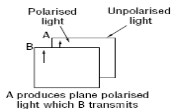• Light source and two pieces of polaroid as shown.
• Rotate one polaroid relative to the other and note that the light intensity transmitted through both polaroids increases and decreases
• Conclusion: Only transverse waves can be polarised, so light is a transverse wave.
•
• Light waves are transverse; sound waves are longitudinal.
• Light waves can be polarised; sound waves cannot be polarised.
• Light waves travel through a vacuum; sound waves cannot travel through a vacuum.
• Dispersion is the breaking up of white light into its constituent colours
• Rainbow / oil film colours / soap bubble colours
•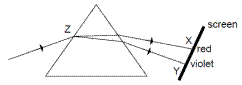A Spectrum is the range of colours present in white light.
• Apparatus: white light source, prism, screen

Procedure: shine light through the prism and rotate the prism.
Observation: different colours are visible on the screen.

•
• It changes direction.
• X = infra-red, Y = ultra-violet
• Red, green, blue
• All colours can be made by mixing red, green and blue.
• Mix two primary colours.
• Complementary colours are pairs of colours consisting of a primary and a secondary colour, such that when combined they give white light.
• Infra-red and ultra-violet
• A = X-rays

• Gamma rays
•
 short wavelength                                                         →                                                 long wavelength gamma rays X-rays ultraviolet light infrared microwaves Radio waves
• Travel at the speed of light, can travel through vacuum, refraction, diffraction, polarisation, interference, etc.
• x-rays, microwaves, ultra-violet etc.
• They travel at speed of light, electromagnetic radiation, travel through vacuum, can be reflected, refracted, polarized etc.
• Temperature sensor, photographic film, blackened thermometer, infrared camera.
• The material fluoresces (glows) when it absorbs white light.
• Radar, mobile phones, speed trap, microwave oven/ cooking, communications /satellite TV / weather radar / missile guidance etc.
• Infra Red: Source of heat, keep things warm, hatch chickens, heat treatment of muscles etc.
• Ultra-violet: detect forged currency, disco lights, used in insect removal device, sterilisation, suntan, forensics, etc.
• To photograph bones/ internal organs, to treat cancer, to detect flaws in materials.
• Electromagnetic waves, have high frequency/ short wavelength, cause ionisation, penetrate materials, no mass and no charge.
• Gammarays / X-rays / UV  - they can all cause ionization of body cells.
• v = s/t

(3.0 × 108) = (1.2 × 1012)/t
t = 4000 s

• c = fλ         Þ f = c/ λ        Þ        f = (3 × 108)/100          Þ       f = 3 × 106 Hz.
• They have a low frequency / long wavelength / low energy.
• λ = c /f       Þ λ = 3.0 × 108 / 252 × 103 = 1.19 × 103 m
• A = (turn)table, B = telescope
• Measure wavelength of light / demonstrate spectra / chemical analysis, etc.
•
• Power = Energy/time = 0.36 / (3 × 60) = 0.002 W

SAR = Power/mass = 0.36/(3 × 60)(10 ×10-3) = 0.20 W kg-1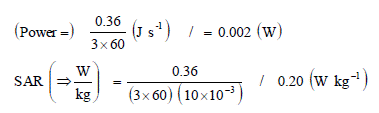• It is converted into heat in the body.
• They have a low frequency / long wavelength / low energy.
• Keep phone at distance, use loudspeaker function, ‘no hands, brief calls only, direct antenna away from your head etc.

Mandatory Experiment

•
• d = 1/80000 = 1.25 × 10-5 m

q = tan-1 (0.238/0.90)
n = 3
nl = d sin q           Þ        l = d sin q/n    Þ l = 551 (± 5) × 10-9 m.

• For maximum number q = 900      Þ sin q = 1

nl = d sin q           Þ nl = d         Þ n = d/l

• n = 22.7 so the greatest whole number of images is 22.

But this is on one side only.
In total there will be 22 on either side, plus one in the middle, so total = 45

• Different colours have different wavelengths so constructive interference occurs at different positions for each separate wavelength.
• At central image q = 0 so constructive interference occurs for all separate wavelengths at the same point so no separation of colours.

•
•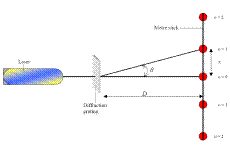See diagram
• The laser
• Distance from grating to screen

Distance between dots on the screen

• 600 lines per mm = 600000 lines per metre.

d = (1/ number of lines per metre) = (1/600000) = 1.67 × 10-6 m.

• Using the formula nλ = d sin q, where d was d was calculated above, n was the order of the dots on either side and q corresponded to the angle shown in the diagram.
• Ensure that the diffraction grating is perpendicular to the (monochromatic) light, use a grating with a large number of lines, ensure D is large, repeat for different orders and take the average, etc.

•
•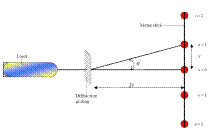A laser or a sodium lamp.
• See diagram.
• Distance from grating to screen

Distance between dots on the screen

• nλ = d sin q
• Ensure that the diffraction grating is perpendicular to the (monochromatic) light, use a grating with a large number of lines, ensure D is large, repeat for different orders and take the average, etc.

•
•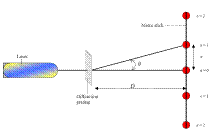See diagram. Plus metre stick
• Nearest on either side of the central (zero order) image.
• Measure x between 1st order images

Measure D from screen to grating
q = tan-1 (x/D)

• Use nλ = d sinθ

(n=1)         λ = sin (17.1)/[(5 × 105)(1)] = 5.8808 × 10-7 ≈ 5.88 × 10-7m
(n=2)         λ = sin (35.8)/[(5 × 105)(2)] = 5.8496 × 10-7 ≈ 5.85 × 10-7m
(n=3)         λ = sin (60.8)/[(5 × 105)(3)] = 5.8195 × 10-7 ≈ 5.82 × 10-7m
λ = 5.85 × 10-7 m = 585 nm

•
• See diagram, plus metre stick.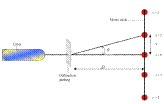Measure distance x from central fringe for n = ±1, ±2
Measure distance D from grating to screen and calculate θ in each case using tan θ = x/D

• nλ = d sinθ

d = 1/500000        Þ        d = 2 × 10-6
n=1, λL= 588.1 nm, λR= 591.4 nm
n=2, λL= 590.6 nm, λR= 592.0 nm
Calculated average wavelength = 590 nm.

• It would result in a smaller value for q which would mean larger percentage errors.
• The grating may not be perpendicular to the incident light

•
• The apparatus was set up as shown.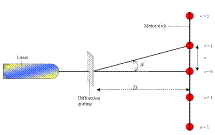To get a value for q the distance x was measured between the centre image and the second order image, then the distance D between grating and screen was measured.
q = Tan-1 (x/D)
We did the same for the other side and got an average value for q.

• Use a laser.
• nλ = d sin θ

n = 2
d = 1/(3.00 x105) m = 3.33 x 10-6 m = 3.33 x 10-3 cm = 1/300 mm
θ = 20.30
λ = 5.78 x 10-7 m (= 578 ≈ 580 nm)

• This would result in a greater angle for each order image and therefore a smaller percentage error in measuring the angle.
• Repeat and get average value for the wavelength , repeat for higher orders.

Source : http://www.thephysicsteacher.ie/LC%20Physics/Student%20Notes/18.%20Light%20Waves.doc

Author : not indicated on the source document of the above text

If you are the author of the text above and you not agree to share your knowledge for teaching, research, scholarship (for fair use as indicated in the United States copyrigh low) please send us an e-mail and we will remove your text quickly.

### The wave nature of light

#### The wave nature of light

Main page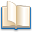Alimentation - nutritionAnimalsArchitectureArtAstrology, esoteric, parapsychology and fantasyAstronomyBiology and geneticsBotany and agricultureChemistryCourses, training, guides, handbooks and tipsCulture General and actualityDermatology, health and wellnessDictionaries and glossariesEconomics and financeEducation and pediatricsEngineering and technologyEnglish grammar and anthologyFashion and show businessFitnessFood, recipes and drinkGames, play spaceGeography, geology and environmentHistoryInformatics and computer worldJobs worldLanguagesLeisure and DIY do it yourselfLessons for studentsLiterature, biographies and journalismMathematics, geometry and statisticalMedicineMusicNotes and concept mapsPhilosophyPhotography and images - picturesPhysicsPhysiologyPsychology and psychoanalysisQuiz questions and answersReligionsRights law and political scienceScienceSociology and cultural anthropologySportSummaries and reviewsTelevision, film - movies and comicsTheater and communicationsTheses, themes and dissertationsTravel and tourist guidesUseful documents and tablesUtility, calculators and convertersWeather and meteorology

#### The wave nature of light

This is the right place where find the answers to your questions like :

Who ? What ? When ? Where ? Why ? Which ? How ? What does The wave nature of light mean ? Which is the meaning of The wave nature of light?

##### The wave nature of light physics notes

Alanpedia.com from 1998 year by year new sites and innovations